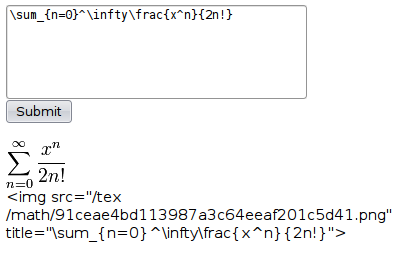# Hex, Bugs and More Physics | Emre S. Tasci

a blog about physics, computation, computational physics and materials…

## LaTeX to PNG and all that equations..

### March 23, 2009 Posted by Emre S. Tasci

After migrating this blog to a new server, I had some difficulties in writing up the equations. It was due to the fact that on this server, I wasn’t able to execute the necessary commands to parse the latex code and convert it to PNG format.

Last week, some need arouse for this and I finally coded the necessary way around to be able to convert LaTeX definitions to their PNG counterparts. Before I start to quote the necessary code and explanations, let me tell that, this method only will work if you can successfully manage to produce this conversation at your -local- computer since what the code does is to generate the image on your local computer and then copy it to the server where your blog (/or whatever you have) resides. So, make sure:

• You can convert LaTeX code to PNG on your -own- computer.
• You are running a httpd server so that the code can copy the output PNG file from your local computer

What the code does:
It has 3 seperate processes. Let’s designate them A, B & C.

• Part A is called on the remote server. It presents a form with textbox in it where you can type the LaTeX code. The form’s target is the same file but this time on your local server, so when you submit the data —
• Part B is called on your local server. Using the texvc code, an open source software that also comes packed with mediawiki, it converts the LaTeX code into a PNG image and places it under the relative math/ directory of your local server. After doing this, via the META tag ‘refresh’ it causes the page to refresh itself loading its correspondence in the remote server, hence initiating —
• Part C where the generated image identified by the MD5 hashname is fetched from the local server and copied unto the relative math/ directory on the remote server. The LaTeX code that was used is also passed in the GET method and it is included in the generated code as the title of the image, so if necessary, the equation can be modified in the future.

The code is as follows:

<?PHP if($_GET["com"]||(!$_POST["formula"] && !$_GET["file"])){?> <body onload="document.postform.formula.focus();"> <form action="http://yourlocalserver.dns.name/tex/index.php" method="post" name=postform id=postform> <textarea name="formula" cols=40 rows=5 id=formula> </textarea><br> <input type="submit" value="Submit" /> <?PHP } if($_POST["formula"])
{
// NL
$lol = './texvc ./mathtemp ./math "'.$_POST["formula"].'" iso-8859-1';
exec($lol,$out);
$file = substr($out,1,32);
$formula = base64_encode($_POST["formula"]);
$html = '<HTML> <HEAD> <META http-equiv="refresh" content="0;URL=http://remoteserver.dns.name/tex/index.php?formula='.$formula.'&file='.$file.'"> </HEAD>'; echo$html;
}
else if($_GET["file"]) { // COM$file = "math/".$_GET["file"].".png"; if(!copy("yourlocalserver.dns.name/tex/".$file, $file)) exit( "copy failed<br>");$formula = base64_decode($_GET["formula"]); ?> <form action="http://yourlocalserver.dns.name/tex/index.php" method="post"> <textarea name="formula" cols=40 rows=5><?PHP echo$formula; ?>
</textarea><br>
<input type="submit" value="Submit" />
<?

echo "<br><br><img src=\"".$file."\" title=\"".$formula."\"><br>";
echo "&lt;img src=\"/tex/".$file."\" title=\"".$formula."\"&gt;<br>";
}
?>


To apply this:

1. Create a directory called ‘tex’ on both servers.
2. Create subdir ‘math’ on both servers
3. Create subdir ‘mathtemp’ on the local server
4. Modify the permissions of these subdirs accordingly
5. Place the executable ‘texvc’ in the ‘tex’ directory
6. Place the code quoted above in the ‘tex’ directories of both servers in a file called ‘index.php’

If everything well up to this point, let’s give it a try and you shall have some output similar to this:For an input LaTeX code of:
\sum_{n=0}^\infty\frac{x^n}{2n!}

where you can immediately see the resulting image, modify the code if there’s something wrong or copy the IMG tag and put it to your raw HTML input box in your text…